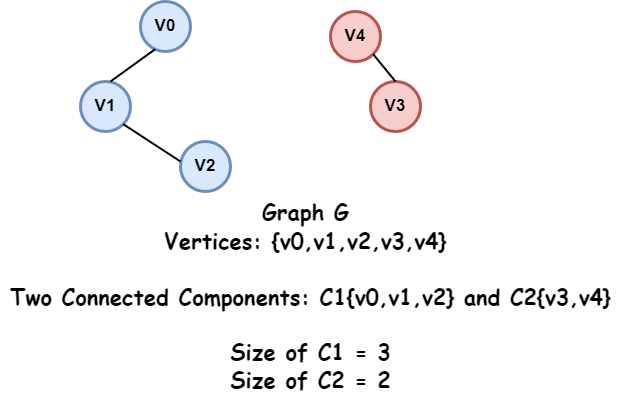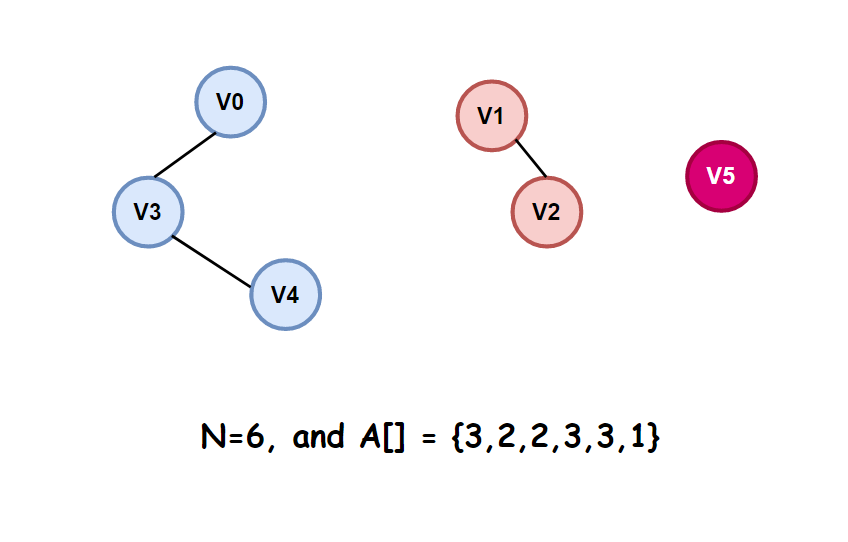# Construct a Graph from the size of components of each node

## Introduction

In this article, we will solve a problem based on graphs and involves a standard concept called connected components in graphs,

If you are not clear about graphs, go through this article to brush up on the important concept - Graph Representation.

Let’s get started with the problem statement.

## Problem Statement

Given an array A[] of size N, the value A[i] such that 0<=i<=N-1 denotes the size of the connected component of the i’th node. You have to find the edges for the possible graph. If not possible to construct a graph following the given condition, print “-1”.

What do you understand by the term “connected component”?

A connected component of an undirected graph is a subgraph in which every pair of nodes is connected by a path formed by the edges.

Now, what is the size of the connected component of the i’th node?

Suppose node i belongs to a component, say C1 of the graph. Then the size of this component is the total number of nodes belonging to this component, including node i.

A picture would make everything clear and easy to understand.The above graph G consists of 5 vertices and 2 connected components. Since component C1 consists of 3 nodes v0, v1 and v2, its size is 3.

What can be the minimum size of a connected component in a graph?

The minimum size of a connected component is 1. Suppose there exists a node that is not connected to any other nodes in the graph. So, it forms a component of size 1.

Let’s walk through an example to solve the problem.

Example:

N=6, and A[] = {3,2,2,3,3,1}

The set of edges of one of the possible graphs that can be constructed is:

``[{1, 2}, {3, 4}, {0, 3}]``

The graph constructed can be shown as:Note that there can be other graphs too satisfying the given conditions.

Like the set of edges - [{1, 2}, {0, 4}, {4, 3}] and [{1, 2}, {0, 4}, {0, 3}] are also valid answers.

Please try to solve this problem on your own before moving on to further discussion here

Let’s see the approach in the next section.

## Approach

First, think of a case where the size of the components of all the nodes are 1.

What does this imply about the graph? It implies there are no edges in the graph, and we do not need to do anything. The output will be an empty list.

Now, the nodes for which A[i]=1 will not form edges as the size of its component is 1. So, we can safely ignore such nodes.

Next comes the real problem. The nodes i, for which A[i] is not equal to 1.

If for node i, the size of its component is A[i]=k, this means that there should be k-1 more nodes having their size of components equal to k.

Now, suppose, for m nodes, the size of their components is k. Then, m must be divisible by k. Why? Because we should be able to group the m nodes into components, each is size k.

Example - if m=4 and k=2 then the vertices v0, v1 form one component and {v2,v3} form other component.

Next, if m=5 and k=2, then we cant divide the 5 vertices into components of size 2 as one component will have only one vertex, so its size will be 2. and hence it's not valid.

We have to do 2 things:

• First, we will check if it is possible to construct a valid graph from the given array A[] by the above condition.
• Then we will proceed to form the edges of a possible graph.

The steps of the implementation of the approach are as follows:

• First, check if the size of components of all the nodes are 1 or not. If all are 1, that means the graph has no edges and we will return an empty list.

• To store the count ‘m’ of all the nodes having the same size of components, we will use a map where the key k is the size of the component and its value is a vector of all nodes i having componentsSize[i]=k.

• Check if a valid graph is possible or not. Iterate over all the elements of the map, and for each element k, get the size m of the vector corresponding to it. If m%k==0, for all elements then a valid graph is possible; else, return -1.

• Initialize a vector of pairs to store the edges of the graph to be constructed.

• Iterate over every element of the map. If there are m nodes in the vector corresponding to component size, we will divide the m nodes into groups of size = component_size to form the graph.

• Compute the number of groups of nodes possible by numGroups = Number of nodes/Component_size.

• For each group, form the edges between the adjacent nodes and push the pair in the vector of edges.

• Finally, print the edges.

In the next section, let’s see the code implementation and the time and space complexity analysis.

## C++ Implementation

``````/*C++ code to construct a Graph from the size of components of each node*/
#include <bits/stdc++.h>
using namespace std;

void graphFromComponentSize(vector<int> &componentsSize, int n)
{
bool flag = false;

unordered_map<int, vector<int>> mp;
for (int i = 0; i < n; i++)//O(n)
{
mp[componentsSize[i]].push_back(i);
if (componentsSize[i] != 1)
flag = true;
}

if (!flag)
{
cout << "No edges exist in the possible graph\n";
return;
}

for (auto a : mp)//O(n)
{
int component_size, num_nodes;
component_size = a.first;
num_nodes = a.second.size();
if (num_nodes % component_size != 0)
{
cout << "No valid graph is possible\n";
return;
}
}

vector<pair<int, int>> edges;

for (auto a : mp)
{
vector<int> nodes = a.second;
int component_size = a.first;

// divide the nodes into groups of size= component_size
int numGroups = nodes.size() / component_size;
for (int i = 0; i < numGroups; i++)
{
int start = i * component_size;
for (int j = start + 1; j < start + component_size; j++)
{
edges.push_back({nodes[j], nodes[j - 1]});
}
}
}

cout << "[";
for (int i = 0; i < edges.size(); i++)
{
cout << "{" << edges[i].first << ", "
<< edges[i].second << "}";
if (i != edges.size() - 1)
{
cout << ", ";
}
}
cout << "]";
}

int main()
{
int n = 6;
vector<int> componentsSize = {3, 2, 2, 3, 3, 1};
graphFromComponentSize(componentsSize, n);

return 0;
}``````

Output

``[{2, 1}, {3, 0}, {4, 3}]``

### Time Complexity

O(n), where n=number of nodes in the graph.

In the function, graphFromComponentSize(), we first iterate over all the nodes to store the values in a map which is an O(n) operation.

Next, we check if a valid graph is possible or not by iterating over all elements of the map, which is also an O(n) operation.

Then, we again iterate through all the map elements to form the edges, and since we iterate through every node only once, its complexity is also O(n).
Hence, the total time complexity is O(n).

### Space Complexity

O(n), where n=number of nodes in the graph. Since we use a map to store the size of a component as a key and vector of nodes corresponding to it.

1. What are graph data structures used for?
Graphs in data structures are used to represent the relationships between objects.

2. What is a connected component in graph theory?
A connected component or simply component of an undirected graph is a subgraph in which each pair of nodes is connected with each other via a path.

3. Which graph traversal can be used to determine the connected components in a graph?
We can use a traversal algorithm, either depth-first or breadth-first, to find the connected components of an undirected graph.

## Key Takeaways

In this article, we discussed an interesting problem based on graphs that are crucial for tech interviews. We solved the problem using a standard concept in graph theory called connected components in an undirected graph.

Before ending this article, some of the follow-up problems that you can solve based on a similar concept are:

If you want to get confident about the various algorithms in graphs, check out Greedy Algorithms in Graph to learn all the important algorithms like Kruskal’s algorithm, Prim’s algorithm etc., on graphs in one place.

Also, practice TOP GRAPHS INTERVIEW QUESTIONS from Codestudio to ace your next technical interview.

Are you planning to ace the interviews of reputed product-based companies like Amazon, Google, Microsoft, and more? Attempt our Online Mock Test Series on CodeStudio now!